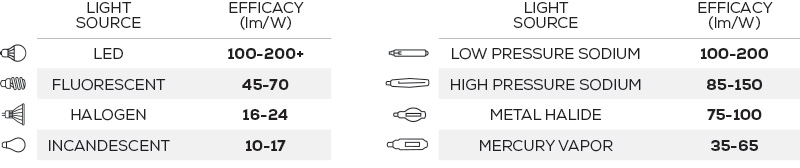## Guide | Power Factor

Posted by Bulb Basics on Jun 27th 2019What Is Power Factor and Why Does It Matter?Power Factor (PF)

PF is used to determine how effectively energy is being utilized. It is measured from −1 to 1 with 1 being the most effective, and is calculated by dividing working power (kW) by apparent power (kVA).Efficacy

Efficacy is measured in lumens per watt and describes the amount of useful light output per unit of electricity. This is calculated by dividing the total lumen output by the total wattage consumed.Efficiency

Efficiency is measured in percentage and is calculated by dividing the total amount of light coming out of the fixture by the amount of light produced by the light source.

Power Factor Beer AnalogyLuminous Efficacies by Light SourceCheck out more of our website at bulbbasics.com